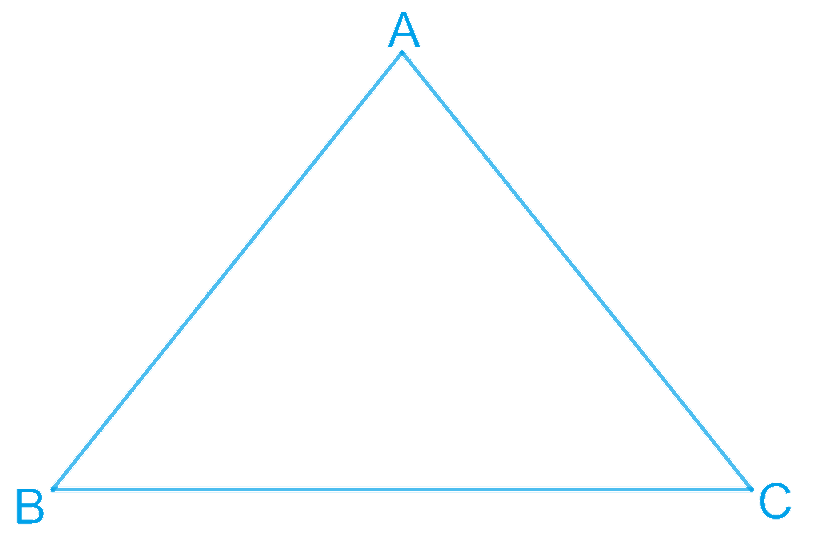In the verge of coronavirus pandemic, we are providing FREE access to our entire Online Curriculum to ensure Learning Doesn't STOP!

# Ex.7.2 Q8 Triangles Solution - NCERT Maths Class 9

Go back to  'Ex.7.2'

## Question

Show that the angles of an equilateral triangle are $$60^{\circ}$$ each.

Video Solution
Triangles
Ex 7.2 | Question 8

## Text Solution

What is Known?

Triangle $$ABC$$ is an equilateral triangle.

To prove:

The angles of an equilateral triangle are $$60º$$ each.

Reasoning:

We can use the property angles opposite to equal sides are equal and then by the angle sum property in triangle $$ABC$$ we can show the value of each angle is $$60$$ degree.

Steps:Let us consider that $$ABC$$ is an equilateral triangle.

Therefore,

\begin{align} &AB=BC=AC \\ &AB=AC \\ \therefore \,&\angle C=\angle B \\ & \left( \begin{array}{I} \text{Angles opposite to equal sides } \\ \text{of a triangle are also equal}\text{.} \\ \end{array} \right) \\ \end{align}

Therefore, we obtain $$\angle A = \angle B = \angle C$$

In $$\Delta ABC,$$
\begin{align} \angle A+\angle B+\angle C&=180^{\circ} \\ \angle A+\angle A+\angle A&=180^{\circ} \\ 3\angle A&=180^{\circ} \\ \angle A&=60^{\circ} \\ \therefore \angle A=\angle B&=\angle C=60^{\circ} \\ \end{align}

Hence, in an equilateral triangle, all interior angles are of measure $$60^{\circ}$$.

Video Solution
Triangles
Ex 7.2 | Question 8

Learn from the best math teachers and top your exams

• Live one on one classroom and doubt clearing
• Practice worksheets in and after class for conceptual clarity
• Personalized curriculum to keep up with school